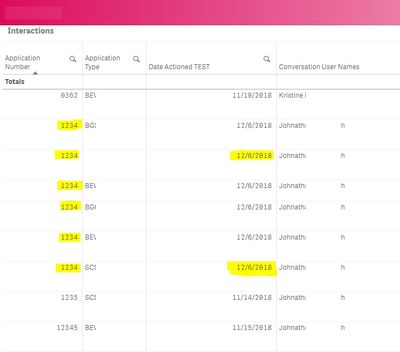# New to Qlik Sense

If you’re new to Qlik Sense, start with this Discussion Board and get up-to-speed quickly.

Announcements
WE ARE LISTENING! New Navigation for Qlik Community, Sept. 26: TELL ME MORE
cancel
Showing results for
Did you mean:Contributor II

## Load only Max Date per Application Number

Hi All,

What expression can I use if I want to show just the Max date for a given dimension (e.g. application number) in QlikSense? Like for every application number who called on different dates, I only want the report to reflect the most recent date it was handled, not considering if it was handled by different agents (or have different application type, please see image for example)?

Here are the expressions I've tried, with not much success:

=aggr(DISTINCT Max(Date(floor((([Date Actioned]))))),[CSD.Application Number])

=Aggr(Max(date(floor([Date Actioned]))), [CSD.Application Number])

=concat({\$<[Date Actioned]={"\$(=max([Date Actioned])"}>} [CSD.Application Number])

=Aggr(distinct(Max(date(floor([Date Actioned])))), [CSD.Application Number])

=FirstSortedValue(Aggr(max(date(floor([Date Actioned])))[CSD.Application Number]))

=Sum({<[Date Actioned]={\$(=Max([Date Actioned]))}>}[CSD.Application Number])

=Only({<[Date Actioned] = {\$(=Date(Max([Date Actioned])))}>} [CSD.Application Number])

Thank you!Labels (6)

• ### unique identifier